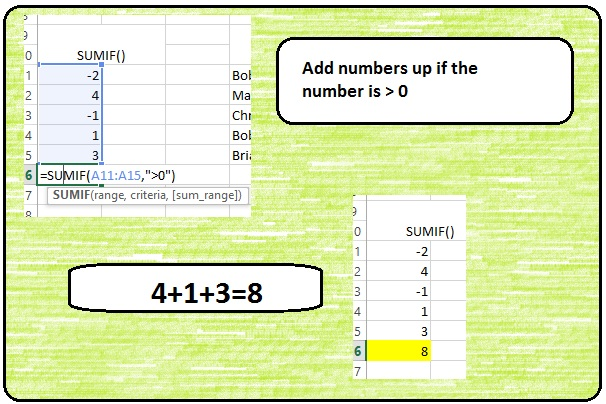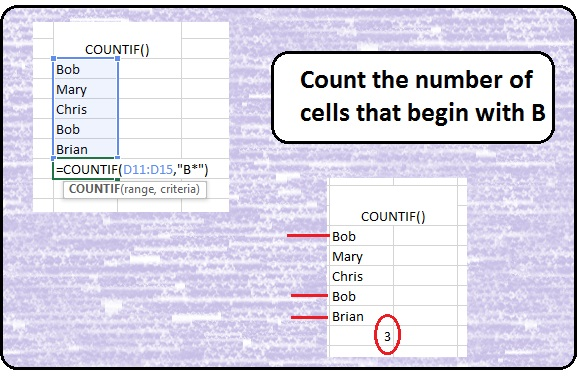# SUMIF() and COUNTIF()

## SUMIF()

SumIf is a handy Excel function to have in your tool box. It is basically a combination of SUM() and IF(). What it is saying is, SUM up all numbers in a range that meet certain condition.

The syntax is as follows:

=SUMIF(range, criteria, [sum_range])

Range = range of numbers you want to test the criteria against

Criteria = the logical test (is the data in the range =, >, < a value)

[sum_range] = this is an optional value. It tells Excel which cells to add. If you don’t use this value, Excel automatic defaults to the first Range## COUNTIF()

CountIF counts the number of cells that meet a certain criteria.

Syntax is similar to SUMIF():

=COUNTIF(range, criteria)

** Note this example uses wildcards – symbols that can be used in place of any symbol. In Excel, the two most popular wildcards are * and ?. ? can be used in place of one character (B?d = Bad, but B?d <> Bead). * can be used in place of many characters. B* = Brain and B*d = Bead)